# 1. Mycat 的分片 join

## 1.1. join 概述

Join 绝对是关系型数据库中最常用一个特性，然而在分布式环境中，跨分片的 join 确是最复杂的，最难解决一个问题。 下面我们简单介绍下各种 Join 操作。

### Inner join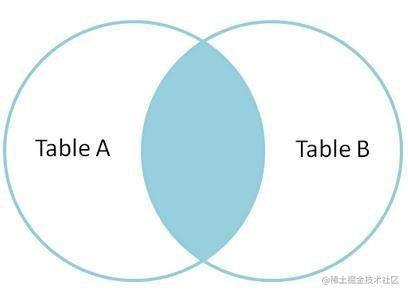### Left join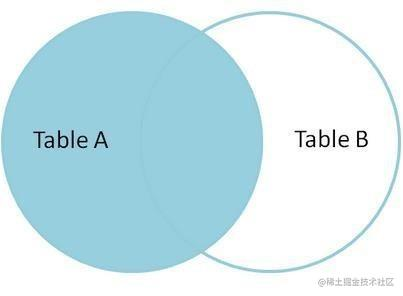### Full join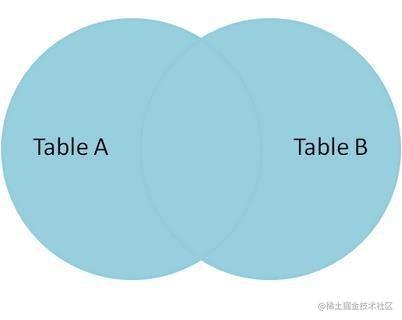### 性能建议

• 尽量避免使用 Left join 或 Right join，而用 Inner join
• 在使用 Left join 或 Right join 时，ON 会优先执行，where 条件在最后执行，所以在使用过程中，条件尽可能的在 ON 语句中判断，减少 where 的执行
• 少用子查询，而用 join。

Mycat 目前版本支持跨分片的 join，主要实现的方式有四种。全局表，ER 分片，catletT(人工智能)和 ShareJoin，ShareJoin 在开发版中支持，前面三种方式 1.3.0.1 支持。

## 1.2. Mycat 的全局表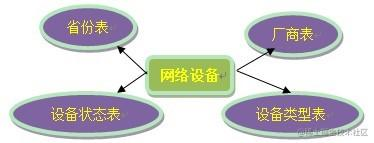• 变动不频繁
• 数据量总体变化不大
• 数据规模不大，很少有超过数十万条记录

• 全局表的插入、更新操作会实时在所有节点上执行，保持各个分片的数据一致性
• 全局表的查询操作，只从一个节点获取
• 全局表可以跟任何一个表进行 JOIN 操作

### 配置

<table name="company" primaryKey="ID" type="global" dataNode="dn1,dn2,dn3" />

## 1.3. ER Join

MyCAT 借鉴了 NewSQL 领域的新秀 Foundation DB 的设计思路，Foundation DB 创新性的提出了 Table Group 的概念，其将子表的存储位置依赖于主表，并且物理上紧邻存放，因此彻底解决了 JION 的效率和性能问题，根据这一思路，提出了基于 E-R 关系的数据分片策略，子表的记录与所关联的父表记录存放在同一个数据分片上。

customer 采用 sharding-by-intfile 这个分片策略，分片在 dn1,dn2 上，orders 依赖父表进行分片，两个表的关联关系为 orders.customer_id=customer.id。于是数据分片和存储的示意图如下：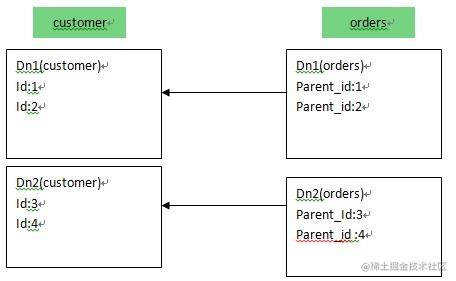### 配置

<table name="customer" dataNode="dn1,dn2" rule="sharding-by-intfile">
<childTable name="orders" joinKey="customer_id" parentKey="id"/>
</table>

## 1.4. Share join

ShareJoin 是一个简单的跨分片 Join，基于 HBT 的方式实现。目前支持 2 个表的 join，原理就是解析 SQL 语句，拆分成单表的 SQL 语句执行，然后把各个节点的数据汇集。

### 配置

A,B 的 dataNode 相同

<table name="A" dataNode="dn1,dn2,dn3" rule="auto-sharding-long" />
<table name="B" dataNode="dn1,dn2,dn3" rule="auto-sharding-long" />

A,B 的 dataNode 不同

<table name="A" dataNode="dn1,dn2 " rule="auto-sharding-long" />
<table name="B" dataNode="dn1,dn2,dn3" rule="auto-sharding-long" />

<table name="A" dataNode="dn1 " rule="auto-sharding-long" />
<table name="B" dataNode=" dn2,dn3" rule="auto-sharding-long" />

## 1.5. catlet（人工智能）

select a.id,a.name,b.title from a,b where a.id=b.id;

    // 包含 MyCat.SQLEngine
EngineCtx ctx = new EngineCtx();
String sql = "select a.id, a.name from a";
// 在 a 表所在的所有分片上顺序执行下面的本地 SQL
ctx.executeNativeSQLSequnceJob(allAnodes, new DirectDBJoinHandler());

DirectDBJoinHandler 类是一个回调类，负责处理 SQL 执行过程中返回的数据包，这里的这个类，主要目的是用 a 表返回的 ID 信息，去 b 表上查询对于的记录，做实时的关联：

    public class DirectDBJoinHandler {

// key 为 id,value 为一行记录的 Column 原始 Byte 数组，
// 这里是 a.id,a.name,b.title 这三个要输出的字段
private HashMap<byte[], byte[]> rows;

// 保存 Header 信息，用于从 Row 中获取 Field 字段值
}

public Boolean onRowData(byte[] rowData) {
String id = getColumnAsString("id");
// 放入结果集,b.title 字段未知，所以先空着
rows.put(getColumnRawBytes("id"), rowData);
// 满 1000 条，发送一个查询请求
String sql = "select b.id, b.name from b where id in(......)";
// 此 SQL 在 B 的所有节点上并发执行，返回的结果直接输出到客户端
ctx.executeNativeSQLParallJob(allBNodes, sql, new MyRowOutPutDataHandler(rows));
}

public Boolean onRowFinished() {
}

public void onJobFinished() {
if (ctx.allJobFinished()) {

}
}

}

    ctx.setJobEventListener(new JobEventHandler() {
public void onJobFinished() {
client.writeRowEndPackage()
}
});

## 1.6. Spark/Storm 对 join 扩展

Mycat 后续的功能会引入 Spark 和 Storm 来做跨分片的 join, 大致流程是这样的在 Mycat 调用 Spark, Storm 的 API, 把数据传送到 Spark，Storm，在 Spark，Storm 进行 join，在把数据传回 Mycat，Mycat 在返回给客户端。# 2. Mycat 的分片规则

## 2.1. 全局表

<table name="t_area" primaryKey="id" type="global" dataNode="dn1,dn2" />

## 2.2. ER 分片表

<table name="order" dataNode="dn$1-32" rule="mod-long"> <childTable name="order_detail" primaryKey="id" joinKey="order_id" parentKey="order_id" /> </table> 复制代码 ## 2.3. 多对多关联 有一类业务场景是 “主表 A+关系表+主表 B”，举例来说就是 会员+订单+商户，对应这类业务，如何切分？ 从会员的角度，如果需要查询会员购买的订单，那按照会员进行切分即可，但是如果要查询商户当天售出的订单，那又需要按照商户做切分，可是如果既要按照会员又要按照商户切分，几乎是无法实现，这类业务如何选择切分规则非常难。目前还暂时无法很好支持这种模式下的 3 个表之间的关联。目前总的原则是需要从业务角度来看，关系表更偏向哪个表，即“A 的关系”还是“B 的关系”，来决定关系表跟从那个方向存储，未来 Mycat 版本中将考虑将中间表进行双向复制，以实现从 A-关系表 以及 B-关系表的双向关联查询如下图所示：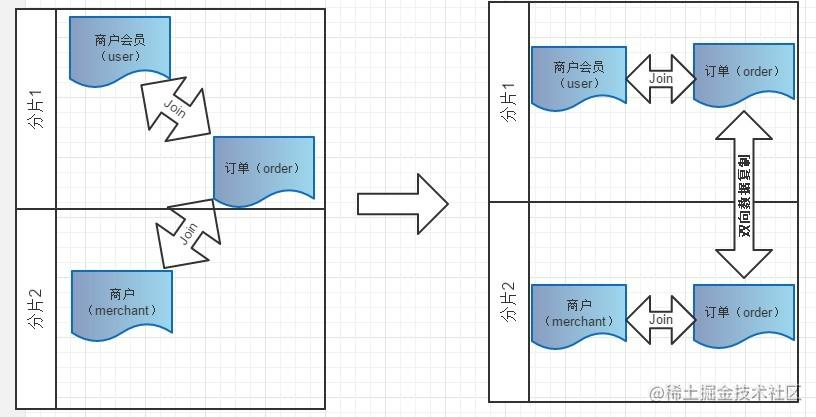### 主键分片 vs 非主键分片 当你没人任何字段可以作为分片字段的时候，主键分片就是唯一选择，其优点是按照主键的查询最快，当采用自动增长的序列号作为主键时，还能比较均匀的将数据分片在不同的节点上。若有某个合适的业务字段比较合适作为分片字段，则建议采用此业务字段分片，选择分片字段的条件如下： • 尽可能的比较均匀分布数据到各个节点上； • 该业务字段是最频繁的或者最重要的查询条件。 常见的除了主键之外的其他可能分片字段有“订单创建时间”、“店铺类别”或“所在省”等。当你找到某个合适的业务字段作为分片字段以后，不必纠结于“牺牲了按主键查询记录的性能”，因为在这种情况下， MyCAT 提供了“主键到分片”的内存缓存机制，热点数据按照主键查询，丝毫不损失性能 <table name="t_user" primaryKey="user_id" dataNode="dn$1-32" rule="mod-long">
<childTable name="t_user_detail" primaryKey="id" joinKey="user_id" parentKey="user_id" />
</table>

## 2.4. Mycat 常用的分片规则

### 2.4.1. 分片枚举

<tableRule name="sharding-by-intfile">
<rule>
<columns>user_id</columns>
<algorithm>hash-int</algorithm>
</rule>
</tableRule>

<function name="hash-int" class="io.mycat.route.function.PartitionByFileMap">
<property name="mapFile">partition-hash-int.txt</property>
<property name="type">0</property>
<property name="defaultNode">0</property>
</function>

partition-hash-int.txt 配置：

10000=0
10010=1
DEFAULT_NODE=1

#### 配置说明

• defaultNode 默认节点：小于 0 表示不设置默认节点，大于等于 0 表示设置默认节点
• 默认节点的作用：枚举分片时，如果碰到不识别的枚举值，就让它路由到默认节点
• 如果不配置默认节点（defaultNode 值小于 0 表示不配置默认节点），碰到不识别的枚举值就会报错

### 2.4.2. 固定分片 hash 算法

<tableRule name="rule1">
<rule>
<columns>user_id</columns>
<algorithm>func1</algorithm>
</rule>
</tableRule>

<function name="func1" class="io.mycat.route.function.PartitionByLong">
<property name="partitionCount">2,1</property>
<property name="partitionLength">256,512</property>
</function>

#### 配置说明

// |<———————1024———————————>|
// |<—-256—>|<—-256—>|<———-512————->|
// | partition0 | partition1 | partition2 |
// | 共 2 份,故 count=2 | 共 1 份，故 count=1 |

int[] count = new int[] { 2, 1 };
int[] length = new int[] { 256, 512 };
PartitionUtil pu = new PartitionUtil(count, length);

<tableRule name="rule1">
<rule>
<columns>user_id</columns>
<algorithm>func1</algorithm>
</rule>
</tableRule>

<function name="func1" class="io.mycat.route.function.PartitionByLong">
<property name="partitionCount">4</property>
<property name="partitionLength">256</property>
</function>

### 2.4.3. 范围约定

• $start <= range <= end$
• $range: start-end$
• $datanode: index$ (K=1000,M=10000)
<tableRule name="auto-sharding-long">
<rule>
<columns>user_id</columns>
<algorithm>rang-long</algorithm>
</rule>
</tableRule>

<function name="rang-long" class="io.mycat.route.function.AutoPartitionByLong">
<property name="mapFile">autopartition-long.txt</property>
<property name="defaultNode">0</property>
</function>

### 2.4.4. 字段取模

<tableRule name="mod-long">
<rule>
<columns>user_id</columns>
<algorithm>mod-long</algorithm>
</rule>
</tableRule>

<function name="mod-long" class="io.mycat.route.function.PartitionByMod">
<!-- how many data nodes -->
<property name="count">3</property>
</function>

### 2.4.5. 按日期（天）分片

<tableRule name="sharding-by-date">
<rule>
<columns>create_time</columns>
<algorithm>sharding-by-date</algorithm>
</rule>
</tableRule>

<function name="sharding-by-date" class="io.mycat.route.function.PartitionByDate">
<property name="dateFormat">yyyy-MM-dd</property>
<property name="sBeginDate">2014-01-01</property>
<property name="sEndDate">2014-01-02</property>
<property name="sPartionDay">10</property>
</function>

#### 配置说明

• columns ：标识将要分片的表字段
• algorithm ：分片函数
• dateFormat ：日期格式
• sBeginDate ：开始日期
• sEndDate：结束日期
• sPartionDay ：分区天数，即默认从开始日期算起，分隔 10 天一个分区如果配置了 sEndDate 则代表数据达到了这个日期的分片后后循环从开始分片插入。
Assert.assertEquals(true, 0 == partition.calculate("2014-01-01"));
Assert.assertEquals(true, 0 == partition.calculate("2014-01-10"));
Assert.assertEquals(true, 1 == partition.calculate("2014-01-11"));
Assert.assertEquals(true, 12 == partition.calculate("2014-05-01"));

### 2.4.6. 取模范围约束

<tableRule name="sharding-by-pattern">
<rule>
<columns>user_id</columns>
<algorithm>sharding-by-pattern</algorithm>
</rule>
</tableRule>

<function name="sharding-by-pattern" class="io.mycat.route.function.PartitionByPattern"
<property name="patternValue">256</property>
<property name="defaultNode">2</property>
<property name="mapFile">partition-pattern.txt</property>
</function>

partition-pattern.txt 配置：

# id partition range start-end, data node index
###### first host configuration
1-32=0
33-64=1
65-96=2
97-128=3
######## second host configuration
129-160=4
161-192=5
193-224=6
225-256=7
0-0=7

#### 配置说明

String idVal = "0";
Assert.assertEquals(true, 7 == autoPartition.calculate(idVal));
idVal = "45a";
Assert.assertEquals(true, 2 == autoPartition.calculate(idVal));

### 2.4.7. 截取数字做 hash 求模范围约束

<tableRule name="sharding-by-prefixpattern">
<rule>
<columns>user_id</columns>
<algorithm>sharding-by-prefixpattern</algorithm>
</rule>
</tableRule>

<function name="sharding-by-prefixpattern" class="io.mycat.route.function.PartitionByPrefixPattern">
<property name="patternValue">256</property>
<property name="prefixLength">5</property>
<property name="mapFile">partition-pattern.txt</property>
</function>

partition-pattern.txt 配置：

# range start-end ,data node index
# ASCII
# 8-57=0-9 阿拉伯数字
# 64、65-90=@、A-Z
# 97-122=a-z

###### first host configuration
1-4=0
5-8=1
9-12=2
13-16=3
###### second host configuration
17-20=4
21-24=5
25-28=6
29-32=7
0-0=7

#### 配置说明

String idVal="gf89f9a";
Assert.assertEquals(true, 0==autoPartition.calculate(idVal));
idVal="8df99a";
Assert.assertEquals(true, 4==autoPartition.calculate(idVal));
idVal="8dhdf99a";
Assert.assertEquals(true, 3==autoPartition.calculate(idVal));

### 2.4.8. 应用指定

<tableRule name="sharding-by-substring">
<rule>
<columns>user_id</columns>
<algorithm>sharding-by-substring</algorithm>
</rule>
</tableRule>

<function name="sharding-by-substring" class="io.mycat.route.function.PartitionDirectBySubString">
<property name="startIndex">0</property><!-- zero-based -->
<property name="size">2</property>
<property name="partitionCount">8</property>
<property name="defaultPartition">0</property>
</function>

### 2.4.9. 截取数字 hash 解析

<tableRule name="sharding-by-stringhash">
<rule>
<columns>user_id</columns>
<algorithm>sharding-by-stringhash</algorithm>
</rule>
</tableRule>
<function name="sharding-by-stringhash" class="io.mycat.route.function.PartitionByString">
<property name="partitionLength">512</property><!-- zero-based -->
<property name="partitionCount">2</property>
<property name="hashSlice">0:2</property>
</function>

#### 配置说明

hashSlice ： 0 代表 str.length(), -1 代表 str.length()-1；

“2” -> (0,2)
“1:2” -> (1,2)
“1:” -> (1,0)
“-1:” -> (-1,0)
“:-1” -> (0,-1)
“:” -> (0,0)

### 2.4.10. 一致性 hash

<tableRule name="sharding-by-murmur">
<rule>
<columns>user_id</columns>
<algorithm>murmur</algorithm>
</rule>
</tableRule>

<function name="murmur" class="io.mycat.route.function.PartitionByMurmurHash">
<property name="seed">0</property><!-- 默认是 0-->
<property name="count">2</property><!-- 要分片的数据库节点数量，必须指定，否则没法分片-->
<property name="virtualBucketTimes">160</property><!-- 一个实际的数据库节点被映射为这么多虚拟

<!--
<property name="weightMapFile">weightMapFile</property>

<!--
<property name="bucketMapPath">/etc/mycat/bucketMapPath</property>

</function>

### 2.4.11. 按单月小时拆分

<tableRule name="sharding-by-hour">
<rule>
<columns>create_time</columns>
<algorithm>sharding-by-hour</algorithm>
</rule>
</tableRule>

<function name="sharding-by-hour" class="io.mycat.route.function.LatestMonthPartion">
<property name="splitOneDay">24</property>
</function>

#### 配置说明

• columns： 拆分字段，字符串类型（yyyymmddHH）
• splitOneDay ： 一天切分的分片数

### 2.4.12. 范围求模分片

<tableRule name="auto-sharding-rang-mod">
<rule>
<columns>id</columns>
<algorithm>rang-mod</algorithm>
</rule>
</tableRule>

<function name="rang-mod" class="io.mycat.route.function.PartitionByRangeMod">
<property name="mapFile">partition-range-mod.txt</property>
<property name="defaultNode">21</property>
</function>

#### 配置说明

partition-range-mod.txt 配置：

0-200M=5  //代表有 5 个分片节点
200M1-400M=1
400M1-600M=4
600M1-800M=4
800M1-1000M=6

### 2.4.13. 日期范围 hash 分片

<tableRule name="rangeDateHash">
<rule>
<columns>col_date</columns>
<algorithm>range-date-hash</algorithm>
</rule>
</tableRule>

<function name="range-date-hash" class="io.mycat.route.function.PartitionByRangeDateHash">
<property name="sBeginDate">2014-01-01 00:00:00</property> <property name="sPartionDay">3</property>
<property name="dateFormat">yyyy-MM-dd HH:mm:ss</property>
<property name="groupPartionSize">6</property>
</function>

sPartionDay 代表多少天分一个分片 groupPartionSize 代表分片组的大小。

### 2.4.14. 冷热数据分片

<tableRule name="sharding-by-date">
<rule>
<columns>create_time</columns>
<algorithm>sharding-by-hotdate</algorithm>
</rule>
</tableRule>

<function name="sharding-by-hotdate" class="io.mycat.route.function.PartitionByHotDate">
<property name="dateFormat">yyyy-MM-dd</property>
<property name="sLastDay">10</property>
<property name="sPartionDay">30</property>
</function>

### 2.4.15. 自然月分片

<tableRule name="sharding-by-month">
<rule>
<columns>create_time</columns>
<algorithm>sharding-by-month</algorithm>
</rule>
</tableRule>

<function name="sharding-by-month" class="io.mycat.route.function.PartitionByMonth">
<property name="dateFormat">yyyy-MM-dd</property>
<property name="sBeginDate">2014-01-01</property>
</function>

#### 配置说明

• columns： 分片字段，字符串类型
• dateFormat ： 日期字符串格式
• sBeginDate ： 开始日期
PartitionByMonth partition = new PartitionByMonth();
partition.setDateFormat("yyyy-MM-dd");
partition.setsBeginDate("2014-01-01");
partition.init();
Assert.assertEquals(true, 0 == partition.calculate("2014-01-01"));
Assert.assertEquals(true, 0 == partition.calculate("2014-01-10"));
Assert.assertEquals(true, 0 == partition.calculate("2014-01-31"));
Assert.assertEquals(true, 1 == partition.calculate("2014-02-01"));
Assert.assertEquals(true, 1 == partition.calculate("2014-02-28"));
Assert.assertEquals(true, 2 == partition.calculate("2014-03-1"));
Assert.assertEquals(true, 11 == partition.calculate("2014-12-31"));
Assert.assertEquals(true, 12 == partition.calculate("2015-01-31"));
Assert.assertEquals(true, 23 == partition.calculate("2015-12-31"));

### 2.4.16. crc32slot 分片算法

crc32solt 是有状态分片算法的实现之一, 数据自动迁移方案设计 crc32(key)%102400=slot;

slot 按照范围均匀分布在 dataNode 上，针对每张表进行实例化，通过一个文件记录 slot 和节点 映射关系，迁移过程中通过 zk 协调其中需要在分片表中增加 slot 字段，用以避免迁移时重新计算，只需要迁移对应 slot 数据即可。分片最大个数为 102400 个，短期内应该够用，每分片一千万，总共可以支持一万亿数据。

<table name="travelrecord" dataNode="dn1,dn2" rule="crc32slot" />

## 2.5. Mycat 多租户支持

1. web 部分修改：

• 修改 jdbc 的实现：在提交 sql 时，从 ThreadLocal 中获取租户 id, 添加 sql 注释，把租户的 schema 放到注释中。例如：/*!mycat : schema = test_01 */ sql ;
2. 在 db 前面建立 proxy 层，代理所有 web 过来的数据库请求。proxy 层是用 mycat 实现的，web 提交的 sql 过来时在注释中指定 schema, proxy 层根据指定的 schema 转发 sql 请求。

3. Mycat 配置：

<user name="mycat">